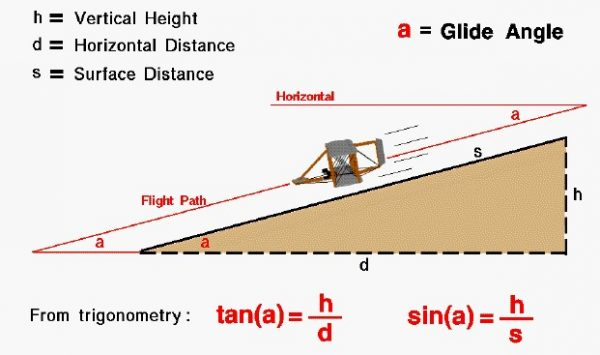# Glide AngleA glider is a special kind of aircraft that has no engine. Paper airplanes are the most obvious example, but gliders come in a wide range of shapes and sizes. Toy gliders, made of balsa wood or styrofoam, are an excellent way for students to study the basics of aerodynamics. The Wright brothers perfected the design of the first airplane and gained piloting experience through a series of glider flights between 1900 and 1902. These aircraft were similar to modern hang-gliders and were launched by running down the side of a sand dune at Kitty Hawk.

## Flight Path

If a glider is in a steady (constant velocity and no acceleration) descent, it loses altitude as it travels. The glider’s flight path is a simple straight line, shown as the inclined red line in the figure. The flight path is inclined to the horizontal at an angle “a” called the glide angle. On another page we will show that the glide angle is related to the ratio of the drag and the lift of the aircraft. The brothers used this knowledge to determine the aerodynamic performance of their gliders. Determining the glide angle in flight is very difficult, so the brothers would fly their glider at a fixed height above the surface of the sand dune. In this flight condition, the flight path is parallel to the surface of the hill and the glide angle is equal to the slope of the hill.

## Sine Angle

The slope of a hill can be easily measured by using a protractor to site the top of the hill from the bottom. If you then measure the distance (s) to the top of the hill by counting paces from the bottom, you can determine the height (h) of the hill by using trigonometry. The height divided by the distance along the surface is equal to the sine (sin) of the measured slope angle (a):

## Tangent Angle

This equation can be solved for the height of the hill. The tangent (tan) of the glide angle (a) is equal to the change in vertical height (h) divided by the horizontal distance (d) flown.

## Angle

If the glider is flown at a specified glide angle, the trigonometric equation can be solved to determine how far the glider can fly for a given change in altitude, or how far it will drop for a given distance flown. Notice that if the glide angle is small, the aircraft can fly a long distance for a small change in altitude. Conversely, if the glide angle is large it can travel only a short distance for a given change in altitude. We can think of the glide angle as a measure of the flying efficiency of the glider. The smaller the glide angle, the farther that an aircraft can fly for a given change in altitude.

Provide feedback